#Function Repository Resource:

# CombinatorEvolve

Evolve a combinator expression for a certain number of steps based on defined rules and an evaluation scheme

Contributed by: Wolfram Research
 ResourceFunction["CombinatorEvolve"][cmb,t] evolves the combinator expression cmb across t steps using S and K combinator transformation rules and single leftmost-outermost updating order. ResourceFunction["CombinatorEvolve"][cmb,t,scheme] evolves cmb using S and K combinator transformation rules in matches found by scheme. ResourceFunction["CombinatorEvolve"][rules,cmb,t,scheme] evolves cmb using rules in matches found by scheme.

## Details and Options

The input cmb must be given as a combinator expression in nested bracket form.
If scheme is specified, direction ("Leftmost", "Rightmost"), depth order ("Outermost", "Innermost") and number of matches (integer number or Infinity) must be specified in order of their priority as sorting criteria.
The combinator transformations of interest should be given as a list of Rule or RuleDelayed elements in rules.
The number of steps t should be an integer number.
ResourceFunction["CombinatorEvolve"] has the following options:
 "MaxSize" maximum leaf count allowed for evolution of cmb "SKGlyphs" symbols used to specify default transformation rules

## Examples

### Basic Examples (2)

Evolve a combinator expression for five steps using the default S and K combinator transformation rules in the single-match leftmost outermost updating scheme:

 In:=Out=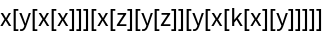Evolve a combinator expression for five steps, specifying that only the S combinator rule should be used in an all-match rightmost-innermost updating scheme:

 In:=Out=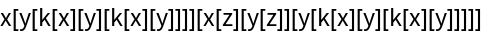### Possible Issues (2)

The "MaxSize" option can be useful for avoiding excessively long combinator evolutions, but will throw a failure if its value is surpassed:

 In:=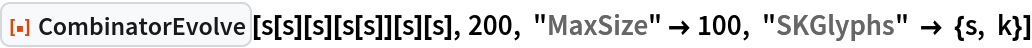Out=CombinatorEvolve returns the fixed-point value if the expression has stopped evolving:

 In:=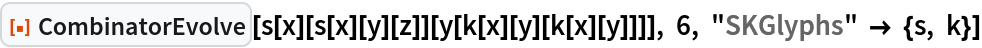Out=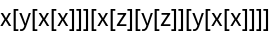In:=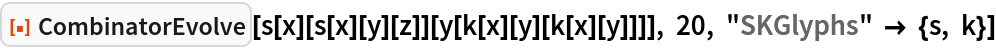Out=